# Shortest Path in Graph

20 Apr 2014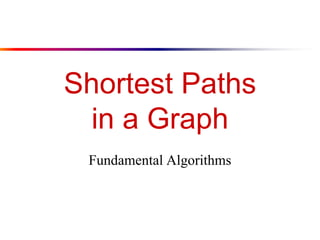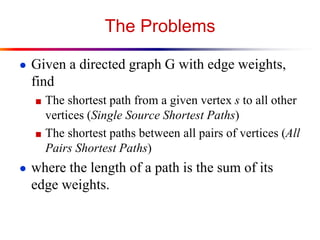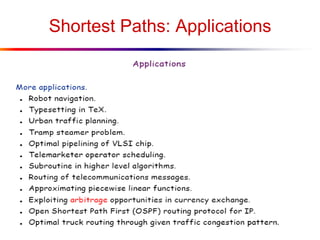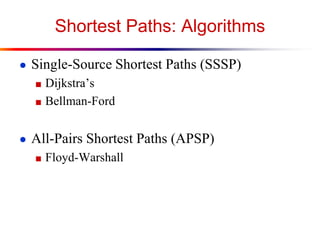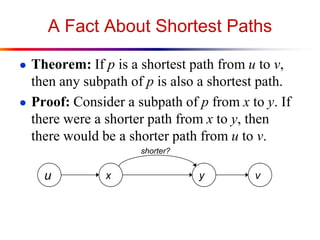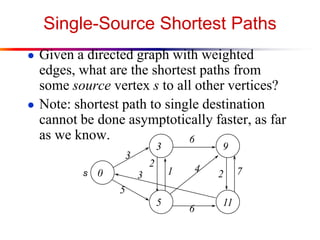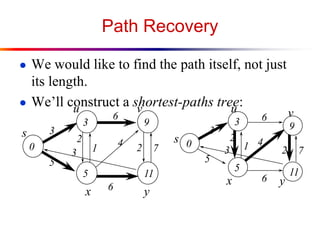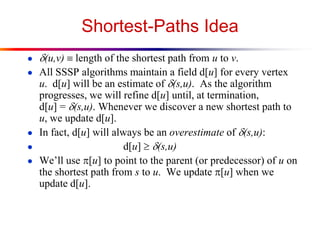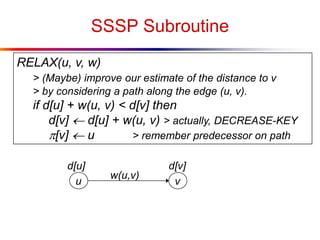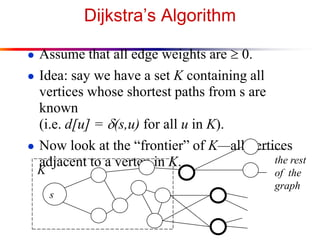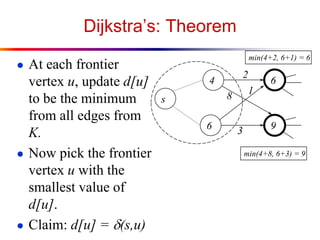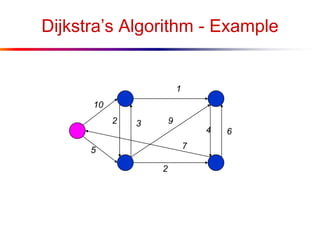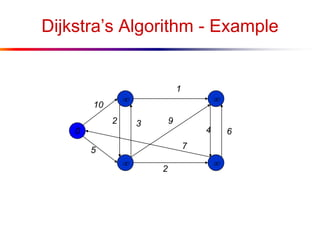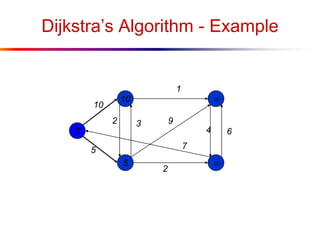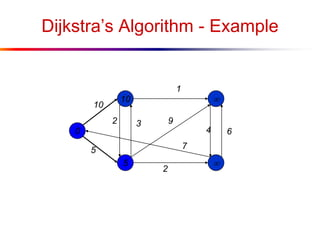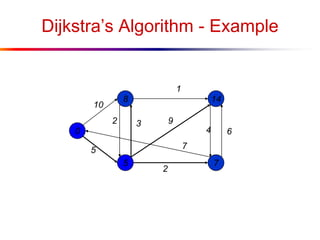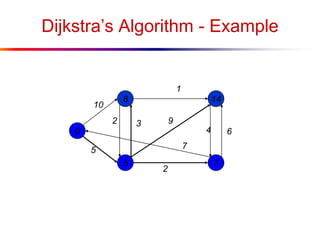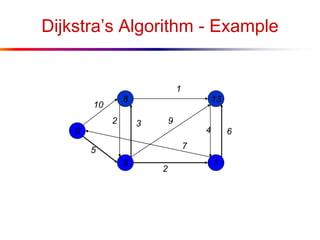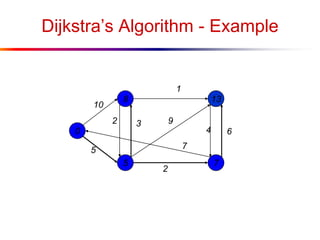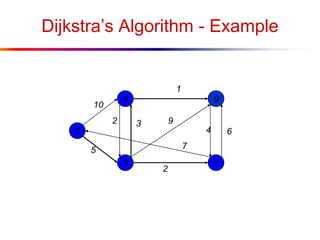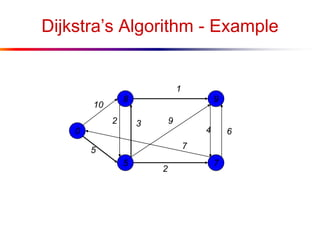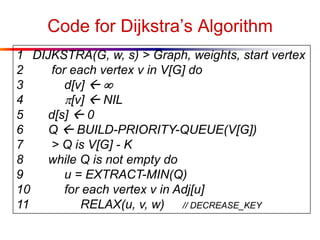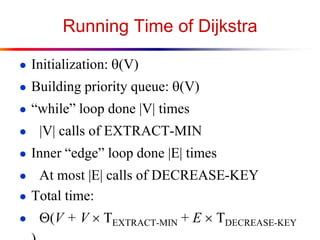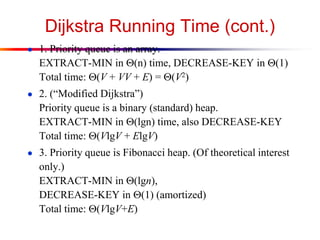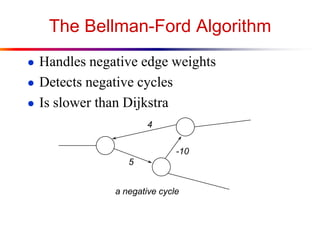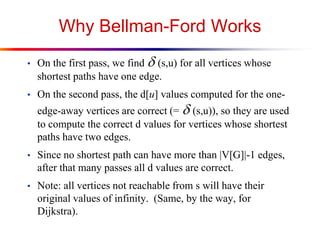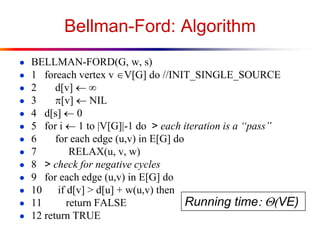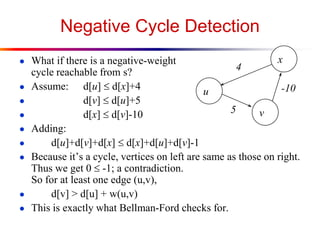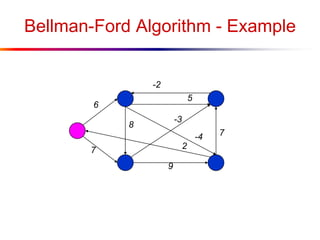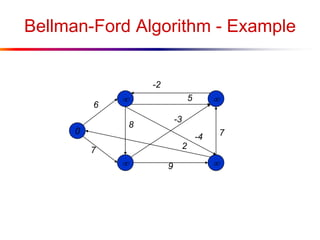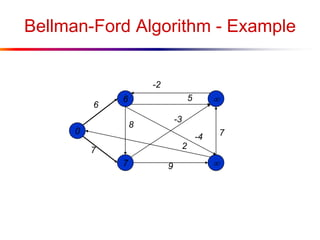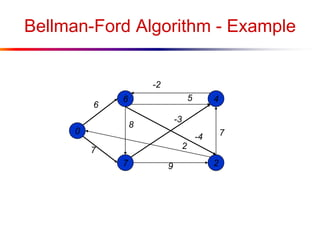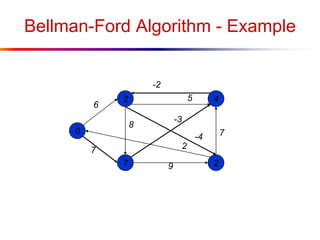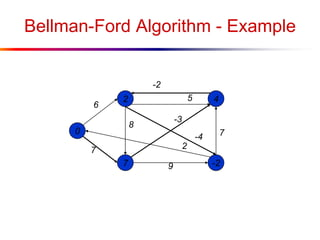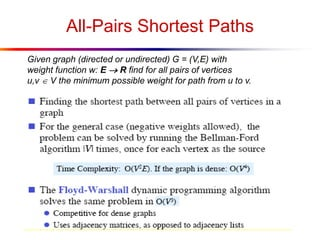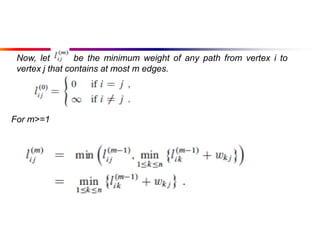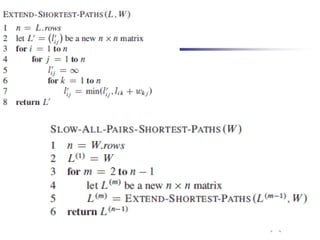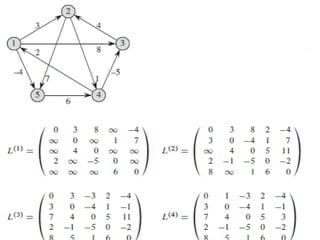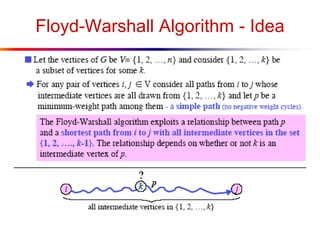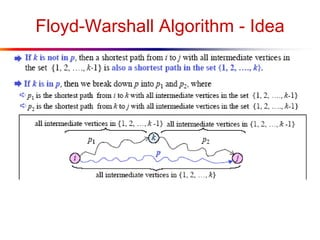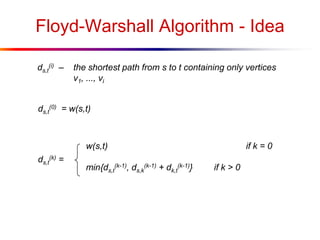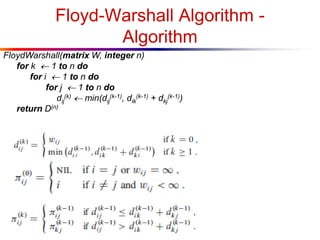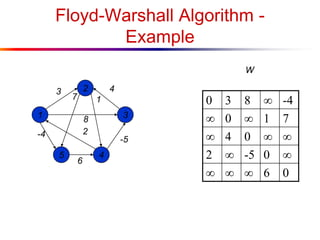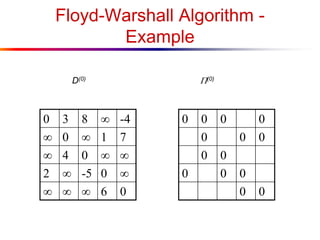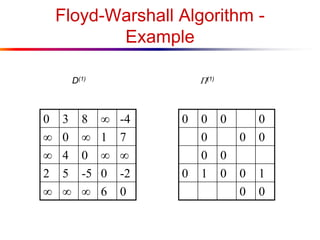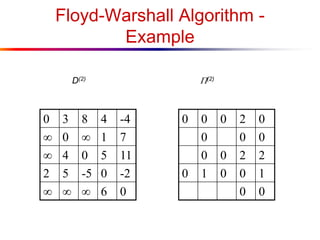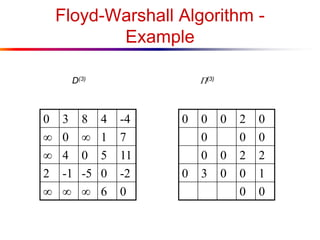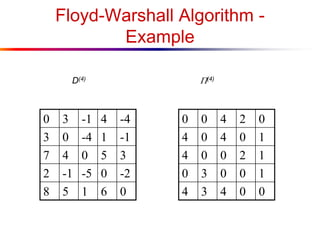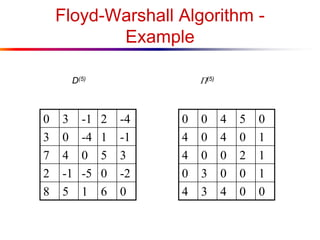1 sur 50

### Shortest Path in Graph

• 1. Shortest Paths in a Graph Fundamental Algorithms
• 2. The Problems ● Given a directed graph G with edge weights, find ■ The shortest path from a given vertex s to all other vertices (Single Source Shortest Paths) ■ The shortest paths between all pairs of vertices (All Pairs Shortest Paths) ● where the length of a path is the sum of its edge weights.
• 4. Shortest Paths: Algorithms ● Single-Source Shortest Paths (SSSP) ■ Dijkstra’s ■ Bellman-Ford ● All-Pairs Shortest Paths (APSP) ■ Floyd-Warshall
• 5. A Fact About Shortest Paths ● Theorem: If p is a shortest path from u to v, then any subpath of p is also a shortest path. ● Proof: Consider a subpath of p from x to y. If there were a shorter path from x to y, then there would be a shorter path from u to v. u x y v shorter?
• 6. Single-Source Shortest Paths ● Given a directed graph with weighted edges, what are the shortest paths from some source vertex s to all other vertices? ● Note: shortest path to single destination cannot be done asymptotically faster, as far as we know. 3 11 9 5 0 3 6 5 4 3 6 2 1 2 7s
• 7. Path Recovery ● We would like to find the path itself, not just its length. ● We’ll construct a shortest-paths tree: 3 11 9 5 0 3 6 5 4 3 6 2 7 2 1 s u v x y 3 11 9 5 0 3 6 5 3 6 2 7 42 1 s u v x y
• 8. Shortest-Paths Idea ● d(u,v)  length of the shortest path from u to v. ● All SSSP algorithms maintain a field d[u] for every vertex u. d[u] will be an estimate of d(s,u). As the algorithm progresses, we will refine d[u] until, at termination, d[u] = d(s,u). Whenever we discover a new shortest path to u, we update d[u]. ● In fact, d[u] will always be an overestimate of d(s,u): ● d[u] d(s,u) ● We’ll use p[u] to point to the parent (or predecessor) of u on the shortest path from s to u. We update p[u] when we update d[u].
• 9. SSSP Subroutine RELAX(u, v, w) > (Maybe) improve our estimate of the distance to v > by considering a path along the edge (u, v). if d[u] + w(u, v) < d[v] then d[v]  d[u] + w(u, v) > actually, DECREASE-KEY p[v]  u > remember predecessor on path u v w(u,v) d[v]d[u]
• 10. Dijkstra’s Algorithm ● Assume that all edge weights are  0. ● Idea: say we have a set K containing all vertices whose shortest paths from s are known (i.e. d[u] = d(s,u) for all u in K). ● Now look at the “frontier” of K—all vertices adjacent to a vertex in K. the rest of the graph s K
• 11. Dijkstra’s: Theorem ● At each frontier vertex u, update d[u] to be the minimum from all edges from K. ● Now pick the frontier vertex u with the smallest value of d[u]. ● Claim: d[u] = d(s,u) s 4 9 6 6 2 1 3 8 min(4+2, 6+1) = 6 min(4+8, 6+3) = 9
• 12. Dijkstra’s Algorithm - Example 10 1 5 2 64 9 7 2 3
• 13. Dijkstra’s Algorithm - Example 0     10 1 5 2 64 9 7 2 3
• 14. Dijkstra’s Algorithm - Example 0 5 10   10 1 5 2 64 9 7 2 3
• 15. Dijkstra’s Algorithm - Example 0 5 10   10 1 5 2 64 9 7 2 3
• 16. Dijkstra’s Algorithm - Example 0 5 8 7 14 10 1 5 2 64 9 7 2 3
• 17. Dijkstra’s Algorithm - Example 0 5 8 7 14 10 1 5 2 64 9 7 2 3
• 18. Dijkstra’s Algorithm - Example 0 5 8 7 13 10 1 5 2 64 9 7 2 3
• 19. Dijkstra’s Algorithm - Example 0 5 8 7 13 10 1 5 2 64 9 7 2 3
• 20. Dijkstra’s Algorithm - Example 0 5 8 7 9 10 1 5 2 64 9 7 2 3
• 21. Dijkstra’s Algorithm - Example 0 5 8 7 9 10 1 5 2 64 9 7 2 3
• 22. Code for Dijkstra’s Algorithm 1 DIJKSTRA(G, w, s) > Graph, weights, start vertex 2 for each vertex v in V[G] do 3 d[v]   4 p[v]  NIL 5 d[s]  0 6 Q  BUILD-PRIORITY-QUEUE(V[G]) 7 > Q is V[G] - K 8 while Q is not empty do 9 u = EXTRACT-MIN(Q) 10 for each vertex v in Adj[u] 11 RELAX(u, v, w) // DECREASE_KEY
• 23. Running Time of Dijkstra ● Initialization: q(V) ● Building priority queue: q(V) ● “while” loop done |V| times ● |V| calls of EXTRACT-MIN ● Inner “edge” loop done |E| times ● At most |E| calls of DECREASE-KEY ● Total time: ● (V + V  TEXTRACT-MIN + E  TDECREASE-KEY
• 24. Dijkstra Running Time (cont.) ● 1. Priority queue is an array. EXTRACT-MIN in (n) time, DECREASE-KEY in (1) Total time: (V + VV + E) = (V2) ● 2. (“Modified Dijkstra”) Priority queue is a binary (standard) heap. EXTRACT-MIN in (lgn) time, also DECREASE-KEY Total time: (VlgV + ElgV) ● 3. Priority queue is Fibonacci heap. (Of theoretical interest only.) EXTRACT-MIN in (lgn), DECREASE-KEY in (1) (amortized) Total time: (VlgV+E)
• 25. The Bellman-Ford Algorithm ● Handles negative edge weights ● Detects negative cycles ● Is slower than Dijkstra 4 5 -10 a negative cycle
• 26. Bellman-Ford: Idea ● Repeatedly update d for all pairs of vertices connected by an edge. ● Theorem: If u and v are two vertices with an edge from u to v, and s  u  v is a shortest path, and d[u] = d(s,u), ● then d[u]+w(u,v) is the length of a shortest path to v. ● Proof: Since s u  v is a shortest path, its length is d(s,u) + w(u,v) = d[u] + w(u,v). 
• 27. Why Bellman-Ford Works • On the first pass, we find d(s,u) for all vertices whose shortest paths have one edge. • On the second pass, the d[u] values computed for the one- edge-away vertices are correct (= d(s,u)), so they are used to compute the correct d values for vertices whose shortest paths have two edges. • Since no shortest path can have more than |V[G]|-1 edges, after that many passes all d values are correct. • Note: all vertices not reachable from s will have their original values of infinity. (Same, by the way, for Dijkstra).
• 28. Bellman-Ford: Algorithm ● BELLMAN-FORD(G, w, s) ● 1 foreach vertex v V[G] do //INIT_SINGLE_SOURCE ● 2 d[v]  ● 3 p[v] NIL ● 4 d[s] 0 ● 5 for i  1 to |V[G]|-1 do > each iteration is a “pass” ● 6 for each edge (u,v) in E[G] do ● 7 RELAX(u, v, w) ● 8 > check for negative cycles ● 9 for each edge (u,v) in E[G] do ● 10 if d[v] > d[u] + w(u,v) then ● 11 return FALSE ● 12 return TRUE Running time:(VE)
• 29. Negative Cycle Detection ● What if there is a negative-weight cycle reachable from s? ● Assume: d[u]  d[x]+4 ● d[v]  d[u]+5 ● d[x]  d[v]-10 ● Adding: ● d[u]+d[v]+d[x]  d[x]+d[u]+d[v]-1 ● Because it’s a cycle, vertices on left are same as those on right. Thus we get 0  -1; a contradiction. So for at least one edge (u,v), ● d[v] > d[u] + w(u,v) ● This is exactly what Bellman-Ford checks for. u x v 4 5 -10
• 30. Bellman-Ford Algorithm - Example 6 7 7 -3 2 8 -4 9 5 -2
• 31. Bellman-Ford Algorithm - Example 0     6 7 7 -3 2 8 -4 9 5 -2
• 32. Bellman-Ford Algorithm - Example 0 7 6   6 7 7 -3 2 8 -4 9 5 -2
• 33. Bellman-Ford Algorithm - Example 0 7 6 2 4 6 7 7 -3 2 8 -4 9 5 -2
• 34. Bellman-Ford Algorithm - Example 0 7 2 2 4 6 5 7 7 -3 2 8 -2 -4 9
• 35. Bellman-Ford Algorithm - Example 0 7 2 -2 4 6 5 7 7 -3 2 8 -2 -4 9
• 36. All-Pairs Shortest Paths Given graph (directed or undirected) G = (V,E) with weight function w: E  R find for all pairs of vertices u,v  V the minimum possible weight for path from u to v.
• 37. Now, let be the minimum weight of any path from vertex i to vertex j that contains at most m edges. For m>=1
• 42. Floyd-Warshall Algorithm - Idea ds,t (i) – the shortest path from s to t containing only vertices v1, ..., vi ds,t (0) = w(s,t) ds,t (k) = w(s,t) if k = 0 min{ds,t (k-1), ds,k (k-1) + dk,t (k-1)} if k > 0
• 43. Floyd-Warshall Algorithm - Algorithm FloydWarshall(matrix W, integer n) for k  1 to n do for i  1 to n do for j  1 to n do dij (k)  min(dij (k-1), dik (k-1) + dkj (k-1)) return D(n)
• 44. Floyd-Warshall Algorithm - Example 2 45 1 3 3 4 -4 -5 6 7 1 8 2 0 3 8  -4  0  1 7  4 0   2  -5 0     6 0 W
• 45. Floyd-Warshall Algorithm - Example 0 3 8  -4  0  1 7  4 0   2  -5 0     6 0 0 0 0 0 0 0 0 0 0 0 0 0 0 0 D(0) (0)
• 46. Floyd-Warshall Algorithm - Example 0 3 8  -4  0  1 7  4 0   2 5 -5 0 -2    6 0 0 0 0 0 0 0 0 0 0 0 1 0 0 1 0 0 D(1) (1)
• 47. Floyd-Warshall Algorithm - Example 0 3 8 4 -4  0  1 7  4 0 5 11 2 5 -5 0 -2    6 0 0 0 0 2 0 0 0 0 0 0 2 2 0 1 0 0 1 0 0 D(2) (2)
• 48. Floyd-Warshall Algorithm - Example 0 3 8 4 -4  0  1 7  4 0 5 11 2 -1 -5 0 -2    6 0 0 0 0 2 0 0 0 0 0 0 2 2 0 3 0 0 1 0 0 D(3) (3)
• 49. Floyd-Warshall Algorithm - Example 0 3 -1 4 -4 3 0 -4 1 -1 7 4 0 5 3 2 -1 -5 0 -2 8 5 1 6 0 0 0 4 2 0 4 0 4 0 1 4 0 0 2 1 0 3 0 0 1 4 3 4 0 0 D(4) (4)
• 50. Floyd-Warshall Algorithm - Example 0 3 -1 2 -4 3 0 -4 1 -1 7 4 0 5 3 2 -1 -5 0 -2 8 5 1 6 0 0 0 4 5 0 4 0 4 0 1 4 0 0 2 1 0 3 0 0 1 4 3 4 0 0 D(5) (5)